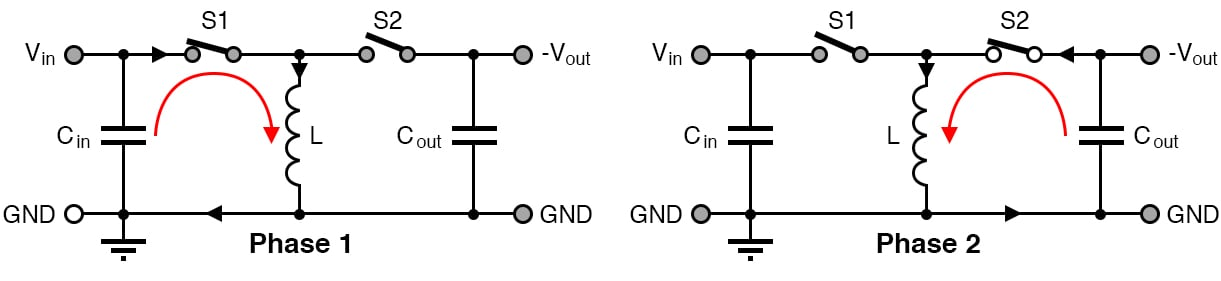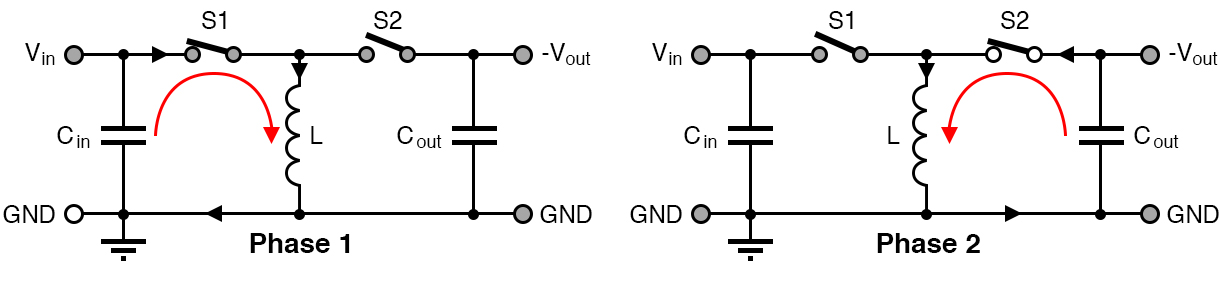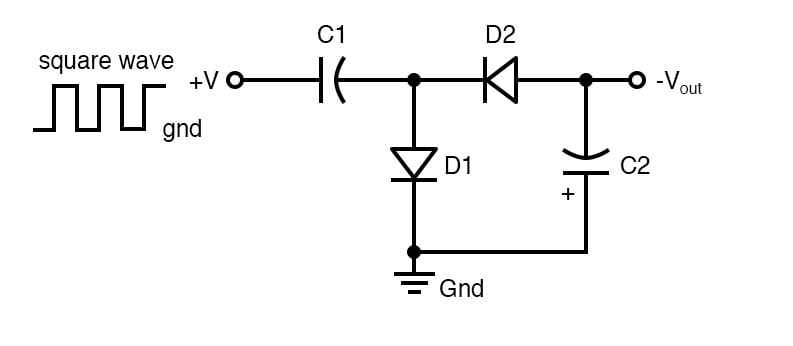Custom

# How to Access Negative Voltage Power SupplyJanuary 07, 2020 by Darshil Patel
ShareLearn about negative voltage, when it's necessary, how to achieve it, and how to make a simple dual-rail supply.

A negative voltage power supply is not the most typical power supply users need but, eventually, everyone needs one.

## What is Negative Voltage?

Before making a negative voltage circuit, we need to understand what a negative voltage is. In a dual rail power supply, it is easy to notice three outputs which are positive, ground and negative voltage.

The ground is the reference voltage for the circuit i.e., 0V. Positive and negative voltages are approximately equal in value but opposite. Opposite in the sense that negative voltage is an excess of electrons and a positive voltage is a deficiency of electrons.

A battery is a very quick negative voltage source. In a 9V battery, there are two terminals: anode for positive voltage and cathode for negative voltage. As most devices are designed for positive voltage supply, the negative terminal serves as a ground. Here when the negative terminal is used as ground, electrons are not returning. Rather, they spread/originate from there. Similarly, when a circuit is designed for negative voltage supply, we can use a cathode as the negative voltage and an anode as ground.

This can be seen with a multi-meter. Check the voltage of the battery with reverse polarity and you can see the same amount of voltage but with a negative sign.

## When is Negative Voltage Used?

For driving devices such as operational amplifiers and transistors, sometimes a negative rail is required. For example, in P channel enhancement type MOSFET, by giving a negative voltage to the base will turn ON the transistor. Many popular chips like LM741 Op-amp. makes use of negative voltage. Here are some more applications where negative voltage is needed:

• In negative voltage regulators
• Building a dual rail power supply
• In audio projects because speakers and piezo elements work great when applied positive and negative voltages.
• In high-performance A/D and D/A converters
• In laser diodes
• LCD bias

## Producing Negative Voltage

One of the other ways to produce a negative voltage is by taking advantage of the effect of capacitive coupling in capacitors.

Capacitive coupling is the effect in which there is the transfer of energy within distant electrical networks via displacement current. Due to this effect, a capacitor tends to keep the voltage across its terminals constant. So when a capacitor's plates are initially 0V and one plate is pulled to 8V, the other plate tries to also rise to 8V. Similarly, when the plate is brought back to 0V, there is a negative charge on the other capacitor plate of -8V.

With this effect in mind, we can now make a negative voltage supply very easily with the help of a couple of switches.Negative voltage power can be achieved with the help of a couple of switches.

In the above circuit, when S1 is closed, Cin charges with the help of positive input voltage. Now when S2 is closed keeping S1 open, Cin discharges to the output capacitor Cout while reversing the polarity. Thus we get a negative voltage at the output.

The above-presented circuit is improved using a simple diode arrangement instead of switches. This circuit is very popularly known as a charge pump circuit.Charge pump circuit

## A Charge Pump Circuit

Here, capacitor C1 charges from 0V to 10V. The negative plate of the capacitor rises from 0V but when it reaches 0.7V, the current is grounded with the help of diode D1. Thus the negative plate of C1 will not be higher than 0.7V. Then when the input voltage drops from 10V to 0V, the negative plate will have 0.7V – 10V = -9.3V of potential difference. D2 is now conducting and we get negative voltage supply at the output.

When this arrangement is connected to an oscillator, a negative voltage is generated at the output keeping C2 charged and thus we get an uninterrupted negative supply.

## Negative Voltage From AC Mains

Getting a negative voltage from the AC power supply is a very easy task; we use the following circuit for this.Circuit for negative voltage from AC mains.

The circuit is built around a transformer, clipping diode and a negative voltage regulator. First of all, we use a 24V transformer to get 24V AC supply. The diode in reverse bias condition acts as a positive clipper. Due to -24 V AC, the large capacitor remains topped up and then for further regulation, we use a 7915 negative voltage regulator IC which will provide us with -15V at the output.

## Dual Rail Power Supply From Batteries

Many a time, one needs a dual rail power supply consisting of +ve, -ve and GND. Making this thing with a battery is a convenient option. We will use a voltage divider circuit for this application and as we know GND is the reference voltage, so we get GND from between the two resistors used as a voltage divider. We get positive voltage supply as Vin/2 and negative voltage of value –Vin/2.Positive and negative voltage with virtual ground

There is one more simple method for getting negative voltage which is by using relays. Relays are very popular in polarity reversal applications and also widely used in motor driving application or driving other actuators. Connecting relays with batteries, we can easily switch between positive and negative voltage.

## A Review of Key Points

We have discussed why negative voltage is important in electronic projects, how to make a simple dual rail power supply, and how to get a negative voltage from:

• A battery
• Charge pump circuits
• AC voltage
• Polarity reversal relays

### AuthorDarshil Patel

I am 19 year old innovator from India currently studying electronics. Am very passionate about electronics since very young age and love to tinker.